#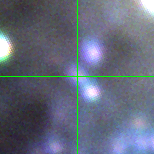V-093765 (J013352.66+303913.9)

## Target Notes### Classification: -

Unresolved from LGGS star of comparable brightness J013352.42+303909.6 (target N093351) 0.24 arcseconds away. All valid measures marked "B" because of unresolved influence. Also pair of LGGS stars 2.2 arcseconds to the NE about 2 magnitudes fainter in all bands. That pair was not resolved properly due in part to the high bacground so it has been ommitted from the PSF fit.

## Plots of Brightness and Color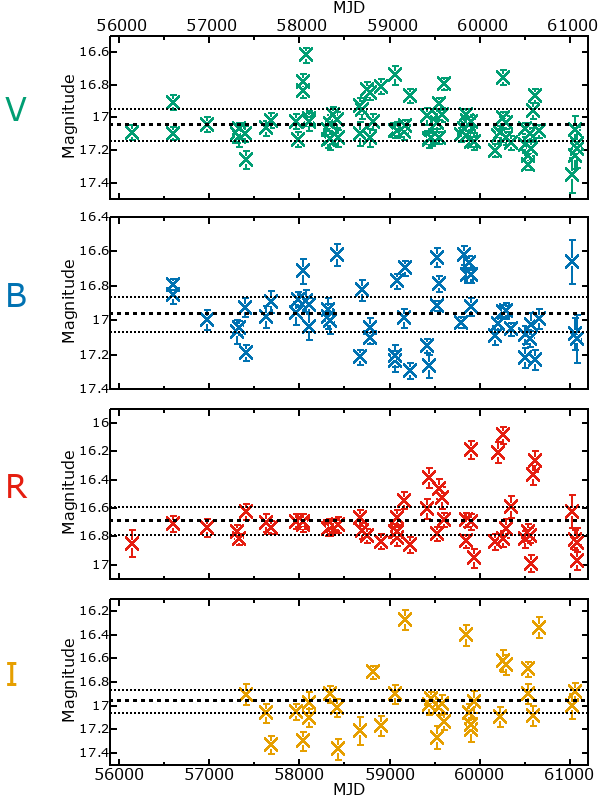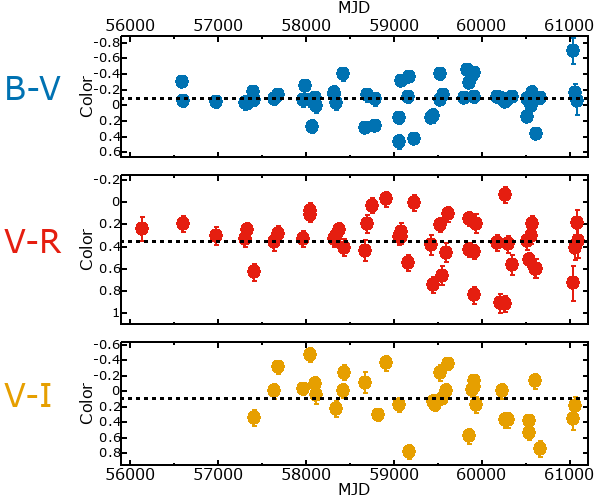## Variability

From the data available in this survey, it is not possible to tell with certainty if this star is variable.

### Correlation factors between bands

If the brightness variation is true variability then there should be a correlation between brightness changes recorded in different filter bands. The following table are the R-squared linear correlation factors calculated for the pairs of bands. The number in parentheses next to the R-squared value is the number of elements used to calculate the correlation factor. The number in square brackets next to the band is the range of magnitudes measured.

V [0.53 mag]B [0.57 mag]R [0.65 mag]
B [0.53 mag]0.02 (29)
R [0.65 mag]0.00 (24)0.00 (16)
I [0.58 mag]0.03 (15)0.19 (9)0.06 (9)

### Magnitude-Magnitude Plots

The plots below are provided to check the values in the table above and also to show any non-linear correlations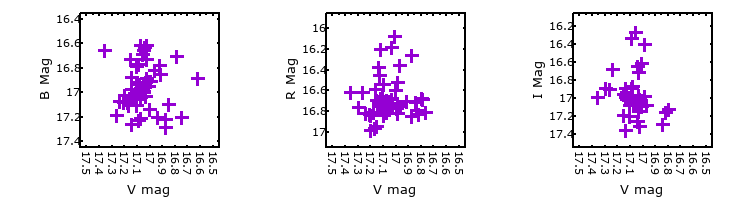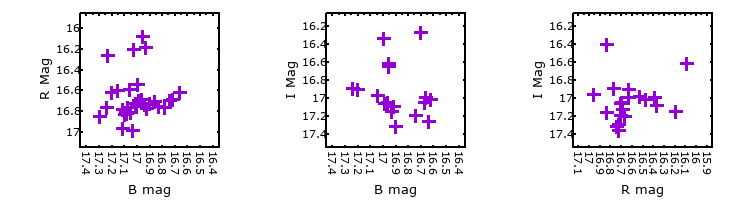### I & R Statistics

Welch & Stetson (1993, AJ, 105, 1813) present a method for varible star detection in CCD images from automated surveys. Data from two bands are compared and two statistics are calculated: R and I.

The I-statistic has an expectation value of zero for stars that are NOT variable. A larger non-zero I-value indicates a higher likelihood that the star is variable. The R statistic indicates how the color of the star changes as the variable grows brighter with R = 1.0 being no change in color.

R and I values have only been calculated if there are more than four (4) matched measurements of brightness in each band.
V
(I|R)
B
(I|R)
R
(I|R)
B
(I|R)
0.91 | 0.88
R
(I|R)
0.69 | 1.100.42 | 0.41
I
(I|R)
0.78 | 0.91-2.36 | 1.48-1.65 | 1.20